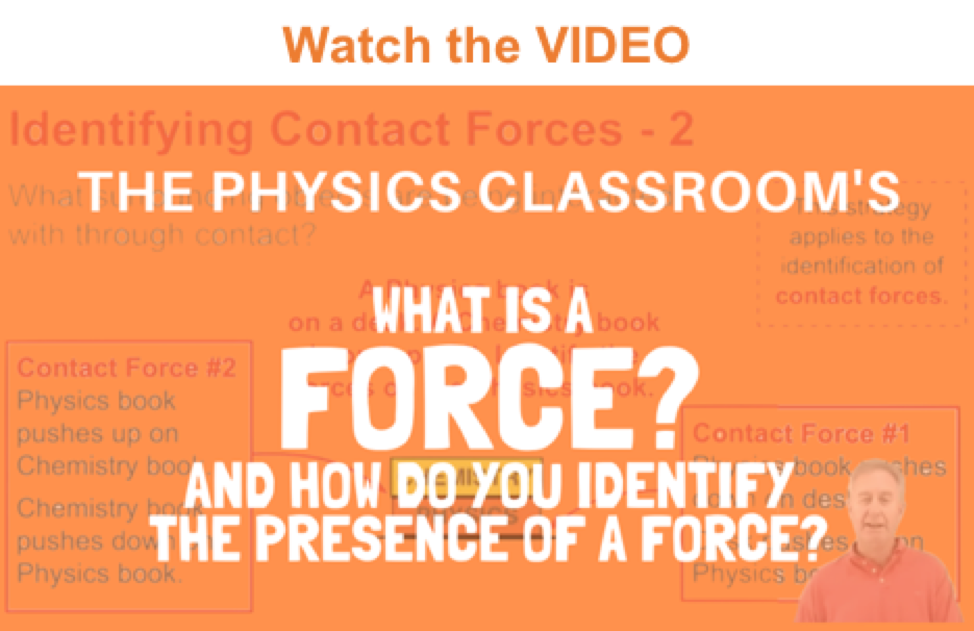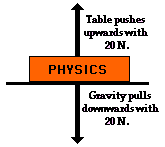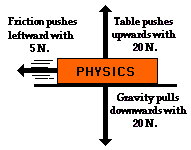Newton's Laws - Lesson 2 - Force and Its Representation

# The Meaning of ForceA force is a push or pull upon an object resulting from the object's interaction with another object. Whenever there is an interaction between two objects, there is a force upon each of the objects. When the interaction ceases, the two objects no longer experience the force. Forces only exist as a result of an interaction.

### Contact versus Action-at-a-Distance Forces

For simplicity sake, all forces (interactions) between objects can be placed into two broad categories:

• contact forces, and
• forces resulting from action-at-a-distance

Contact forces are those types of forces that result when the two interacting objects are perceived to be physically contacting each other. Examples of contact forces include frictional forces, tensional forces, normal forces, air resistance forces, and applied forces. These specific forces will be discussed in more detail later in Lesson 2 as well as in other lessons.

Action-at-a-distance forces are those types of forces that result even when the two interacting objects are not in physical contact with each other, yet are able to exert a push or pull despite their physical separation. Examples of action-at-a-distance forces include gravitational forces. For example, the sun and planets exert a gravitational pull on each other despite their large spatial separation. Even when your feet leave the earth and you are no longer in physical contact with the earth, there is a gravitational pull between you and the Earth. Electric forces are action-at-a-distance forces. For example, the protons in the nucleus of an atom and the electrons outside the nucleus experience an electrical pull towards each other despite their small spatial separation. And magnetic forces are action-at-a-distance forces. For example, two magnets can exert a magnetic pull on each other even when separated by a distance of a few centimeters. These specific forces will be discussed in more detail later in Lesson 2 as well as in other lessons.

Examples of contact and action-at-distance forces are listed in the table below.

 Contact Forces Action-at-a-Distance Forces Frictional Force Gravitational Force Tension Force Electrical Force Normal Force Magnetic Force Air Resistance Force Applied Force Spring Force

### The Newton

Force is a quantity that is measured using the standard metric unit known as the Newton. A Newton is abbreviated by an "N." To say "10.0 N" means 10.0 Newton of force. One Newton is the amount of force required to give a 1-kg mass an acceleration of 1 m/s/s. Thus, the following unit equivalency can be stated:

1 Newton = 1 kg • m/s2

### Force is a Vector Quantity

A force is a vector quantity. As learned in an earlier unit, a vector quantity is a quantity that has both magnitude and direction. To fully describe the force acting upon an object, you must describe both the magnitude (size or numerical value) and the direction. Thus, 10 Newton is not a full description of the force acting upon an object. In contrast, 10 Newton, downward is a complete description of the force acting upon an object; both the magnitude (10 Newton) and the direction (downward) are given.Because a force is a vector that has a direction, it is common to represent forces using diagrams in which a force is represented by an arrow. Such vector diagrams were introduced in an earlier unit and are used throughout the study of physics. The size of the arrow is reflective of the magnitude of the force and the direction of the arrow reveals the direction that the force is acting. (Such diagrams are known as free-body diagrams and are discussed later in this lesson.) Furthermore, because forces are vectors, the effect of an individual force upon an object is often canceled by the effect of another force. For example, the effect of a 20-Newton upward force acting upon a book is canceled by the effect of a 20-Newton downward force acting upon the book. In such instances, it is said that the two individual forces balance each other; there would be no unbalanced force acting upon the book.Other situations could be imagined in which two of the individual vector forces cancel each other ("balance"), yet a third individual force exists that is not balanced by another force. For example, imagine a book sliding across the rough surface of a table from left to right. The downward force of gravity and the upward force of the table supporting the book act in opposite directions and thus balance each other. However, the force of friction acts leftwards, and there is no rightward force to balance it. In this case, an unbalanced force acts upon the book to change its state of motion.

The exact details of drawing free-body diagrams are discussed later. For now, the emphasis is upon the fact that a force is a vector quantity that has a direction. The importance of this fact will become clear as we analyze the individual forces acting upon an object later in this lesson.

Next Section: